#### QuantumField

##### Description

QuantumField is the head of quantized fields and their derivatives. QuantumField[par, ftype, {lorind}, {sunind}] denotes a quantum field of type ftype with (possible) Lorentz-indices lorind and SU(N)-indices sunind. The optional first argument par denotes a partial derivative acting on the field.

##### Examples

This denotes a scalar field.

``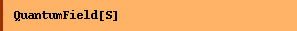``
`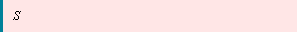`
``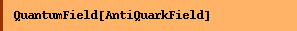``
`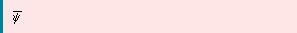`
``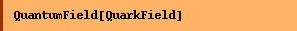``
`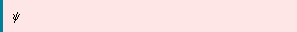`

This is a field with a Lorentz index.

``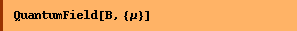``
`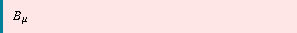`

Color indices should be put after the Lorentz ones.

``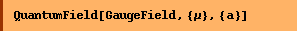``
`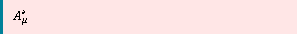`
``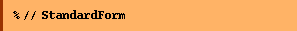``
`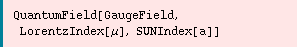`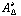is a short form for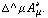``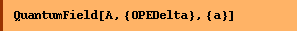``
`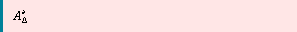`

The first list of indices is usually interpreted as type LorentzIndex, except for OPEDelta, which gets converted to type Momentum.

``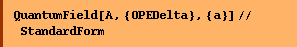``
`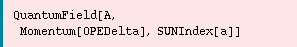`

Derivatives of fields are denoted as follows.

``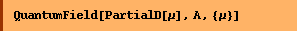``
`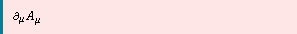`
``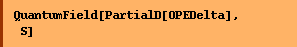``
`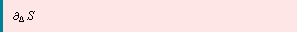`
``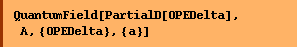``
`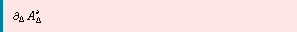`
``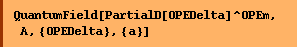``
`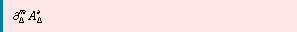`
``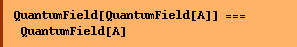``
`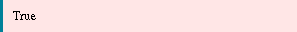`

Converted from the Mathematica notebook QuantumField.nb